Latest Banking jobs   »

# Quantitative Aptitude Quiz For Bank of Baroda AO 2023 -27th April

Direction (1-5): Given below in each question two quadratic equations are given. Please solve and choose the correct option.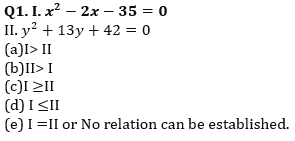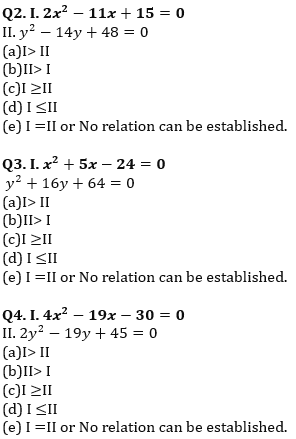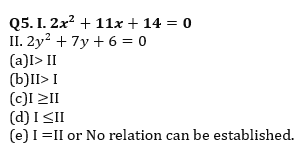Directions (6-15): In each of these questions, two equation (I) and (II) are given. You have to solve both the equations and give answer.
(a) If x>y
(b) If x≥y
(c) If x<y
(d) If x≤y
(e) If x = y or no relation can be established between x and y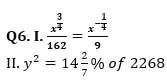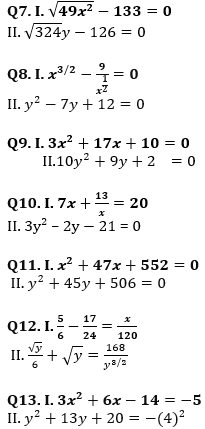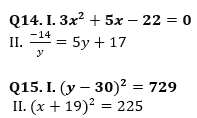Solutions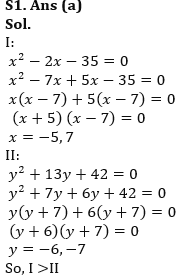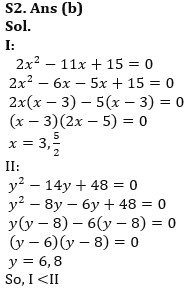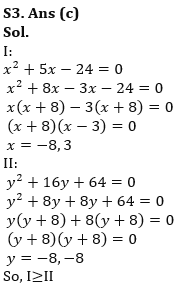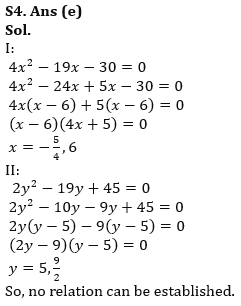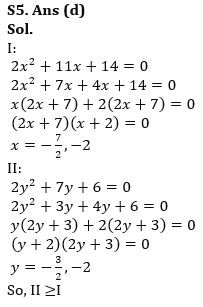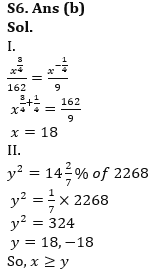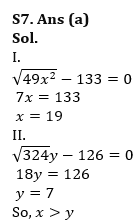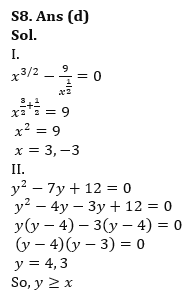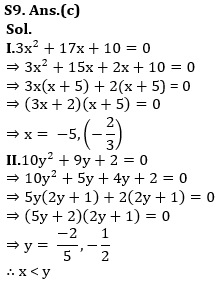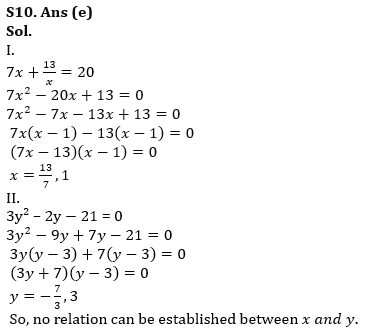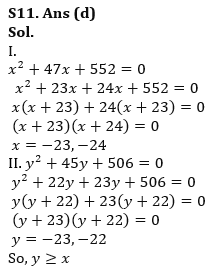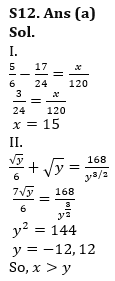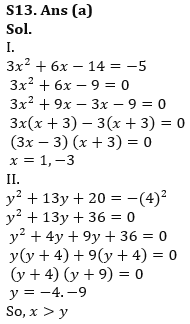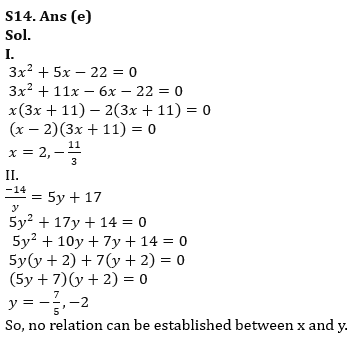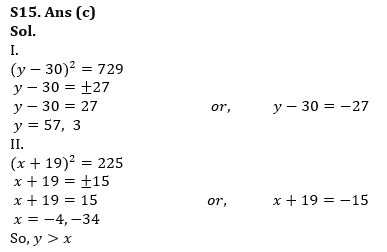…                                                                                                                                                               .

## FAQs

### What is the selection process of Bank of Baroda AO 2023?

The selection process of Bank of Baroda AO consists of Online exam and Interview

#### Congratulations!Union Budget 2023-24: Free PDF